# Smooth supersaturated models

## Smooth supersaturated models

Smooth supersaturated models (SSM) are polynomial models with more terms than there are design points, with the model coefficients selected as thoe that produce the model that minimizes the roughness. Formally, let $$d=(d_1, \dotsc, d_n)^T$$ be a vector of n design points (with no replicated points) in $$d$$ factors and let $$y=(y_1, \dotsc, y_n)^T$$ be a vector of associated observations. Let $$f(x)$$ be an $$N \times 1$$ vector containing a set of linearly independent polynomials with $$N > n$$. The smooth supersaturated model is defined as $s(x)=f(x)^T\theta,$ with $$\theta$$ being the coefficient vector such that for all $$i\in 1,\dotsc,n$$ we have $$s(x_i)=y_i$$ and $\int_{\mathcal X}\sum_{i,j}\frac{\partial s(x)}{\partial x_i \partial x_j}^2~\mathrm dx$ is minimised.

Smooth supersaturated models are are spline-like models and converge to splines as $$N\rightarrow\infty$$. The polynomial nature of the model means that sensitivity indices are computed analytically from the coefficient vector $$\theta$$.

The SSM package defines an S4 class called "SSM" which contains all the information regarding the model basis $$f(x)$$, the coefficient vector $$\theta$$ as well as numerous other information regarding the model such as sensititivity indices and estimated metamodel error. The main function provided by the SSM package is fit.ssm and this is used to construct smooth supersaturated models and analyze them as follows:

design <- seq(-1, 1, 0.25)
responses <- sapply(design, "^", 2)
s <- fit.ssm(design, responses)
s
## Smooth supersaturated model in d=1 variables with N=29 terms over design of n=9 points.
## Smoothness measure Psi_2=7.423354

Also included are methods to plot SSM and predict with SSM.

predict(s, 0.5)
##  0.25
plot(s)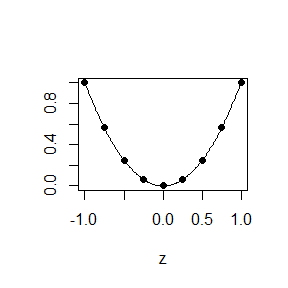Additionally, the sensitivity.plot function is useful for visually identifying important variables and interactions in the model. The transform11 function is used to transform designs to lay within $$\left[-1, 1\right]^d$$, which is assumed by the default behaviour of fit.ssm.

## fit.ssm

In this section we discuss the use of the fit.ssm function. At it’s most basic level it only needs to be supplied with a design – a matrix with each design point being a row, or a vector for one factor data – and a matching vector of responses. It will then generate an appropriately sized basis and fit a model smoothing over $$\left[-1, 1\right]^d$$.

The behaviour of fit.ssm is highly customisable. We highlight the four most useful options:

• basis_size: This option sets the size $$N$$ of the generated basis. By default, when supplied with a dataset of $$n$$ points in $$d$$ factors, fit.ssm will generate a basis with $$N = 20 \times d + n$$ polynomial terms. Larger values of $$N$$ will produce smoother models and are therefore desirable. However, large values of $$N$$ come at a computational cost so the default $$N$$ is rather conservative. Also, values too large will result in numeric instability. It is recommended to plot SSM to check if there are artifacts around the smoothing region border and reducing $$N$$ if problems are present.
# default behaviour
s   <- fit.ssm(design, responses); s
## Smooth supersaturated model in d=1 variables with N=29 terms over design of n=9 points.
## Smoothness measure Psi_2=7.423354
# too large to fit
s100 <- fit.ssm(design, responses, basis_size = 100); s100
## Warning: Unable to fit model due to numeric instability.
## Try reducing the basis size, which is currently 100TRUE
## Smooth supersaturated model in d=1 variables with N=100 terms over design of n=9 points.
## No SSM has been fit.
# instabilty indicated by plot
s70 <- fit.ssm(design, responses, basis_size = 70); s70
## Smooth supersaturated model in d=1 variables with N=70 terms over design of n=9 points.
## Smoothness measure Psi_2=3.42958
plot(s70, main = "70 terms")

s50 <- fit.ssm(design, responses, basis_size = 50); s50
## Smooth supersaturated model in d=1 variables with N=50 terms over design of n=9 points.
## Smoothness measure Psi_2=5.452904
plot(s50, main = "50 terms")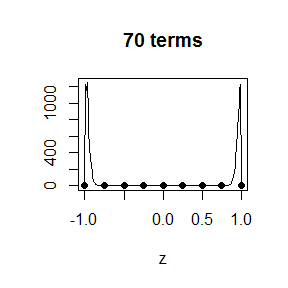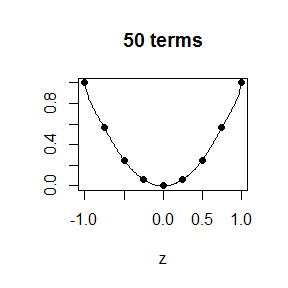• SA: If SA is set to TRUE, then sensitivity indices will be computed for the fitted model. This process is based on the FANOVA decompostition of the model as $s\left(x\right)=s_0+\sum_{i_1=1}^ds_{i_1}\left(x_{i_1}\right)+\sum_{i_1=1}^d\sum_{i_2=i_1+1}^ds_{i_1i_2}\left(x_{i_1}, x_{i_2}\right)+\cdots+s_{i_1\dotsc i_d}\left(x_{i_1},\dotsc,x_{i_d}\right),$ where the terms are orthogonal with respect to some probability measure. The default Legendre polynomials are orthogonal with respect to a uniform measure over $$\left[-1, 1\right]^d$$. Given a subset of variables $$I = \left\{x_{i_1}, \dotsc, x_{i_m}\right\}$$, integrating $$s_{i_1\dots\i_m}^2$$ with respect to the measure gives the associated variance $$D_I$$. The proportion of total variance associated with a given variable or set of variables is known as the Sobol index $$S_I$$. The polynomial nature of SSM provides an analytic formula for these Sobol indices. Also computed are Total indices which are the sum of all $$S_J$$ for which $$I\subset J$$. Printing an SSM object displays the Sobol indices and Total indices for main effects only. Sobol indices are computed for all possible interactions when $$d<11$$ and are stored within the SSM object - see the documentation for more information. Total interaction indices are a special case of Total index where $$\lvert I\rvert = 2$$ and are useful for identifying when interactions are present in the model. The sensitivity.plot function provides useful ways of visualising the sensitivity indices.
f <- function(x) sum(x * 1:3) + 5 * x*x
design <- matrix(runif(300, -1, 1), ncol = 3)
response <- apply(design, 1, f)
s <- fit.ssm(design, response, SA = TRUE)
##
## Computing Sobol indices assuming inputs are uniformly distributed
##         over [-1,1]^d using Legendre polynomials.
##
## Computing main effects.
##
## Computing total effects.
##
## Computing total interaction indices.
##
## Computing Sobol indices for all order interactions.
## Computing total interaction indices.
s
## Smooth supersaturated model in d=3 variables with N=160 terms over design of n=100 points.
## Smoothness measure Psi_2=386.5796
## Main effect Sobol' indices:
##  0.046 0.180 0.407
## Total indices:
##  0.413 0.547 0.407
sensitivity.plot(s, "main_sobol", cex.main = 0.5)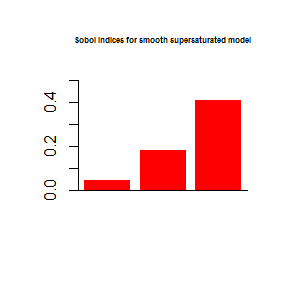# The grey bars indicate interactions
sensitivity.plot(s, "sobol", cex.main = 0.5)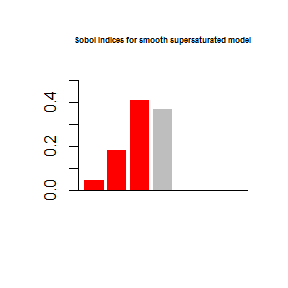# This plots total indices for main effects, and total interaction indices for second order interactions
sensitivity.plot(s, "total", cex.main = 0.5)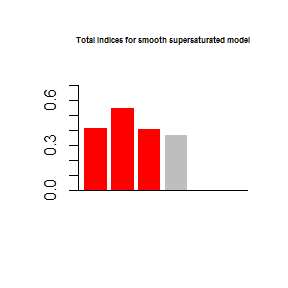If sensitivity analysis indicates that certain interactions of main effects are unimportant, it is possible to fit a new SSM while excluding particular effects and interactions. To do this, pass a list of vectors to the exclude argument. The vector c(1, 2) is associated with the interaction between $$x_1$$ and $$x_2$$ for example, so exclude = list(c(1,2)) will leave out all polynomials which are dependent on $$x_1$$ and $$x_2$$ only.

# A stupid example, but fit new model without main effect of first variable
s3 <- fit.ssm(design, response, SA = TRUE, exclude = list(1))
##
## Computing Sobol indices assuming inputs are uniformly distributed
##         over [-1,1]^d using Legendre polynomials.
##
## Computing main effects.
##
## Computing total effects.
##
## Computing total interaction indices.
##
## Computing Sobol indices for all order interactions.
## Computing total interaction indices.
s3
## Smooth supersaturated model in d=3 variables with N=160 terms over design of n=100 points.
## Smoothness measure Psi_2=27535.96
## Main effect Sobol' indices:
##  0.00 0.18 0.28
## Total indices:
##  0.511 0.691 0.421
sensitivity.plot(s3, "sobol", cex.main = 0.5)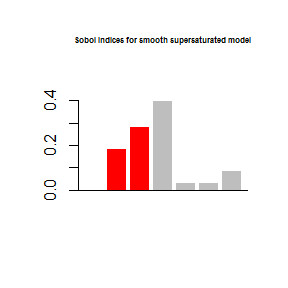It is possible to specify a user-defined polynomial basis by using the basis, P and K options in fit.ssm. If this is done then the sensitivity analysis will assume that the basis is orthonormal with respect to some probability measure which will imply the input distribution. For example, a basis of normalised Hermite polynomials implies that the input distribution is normally distributed with zero mean and unit variance.

• validation: If this is set to TRUE then Leave-One-Out errors will be computed for each design point and the root mean square error (standardised against the variance of the $$\bar y$$ estimator) is calculated.
s <- fit.ssm(design, response, validation = TRUE)
s
## Smooth supersaturated model in d=3 variables with N=160 terms over design of n=100 points.
## Smoothness measure Psi_2=386.5796
## Standardised LOO RMSE = 0.01375434
• GP: If this is set to TRUE then the metamodel error is estimated using a Gaussian process procedure. This functionality is experimental in nature and should be used with caution.The credible intervals of the SSM-GP model tend to be more conservative than kriging models. By default a squared exponential correlation function is used, but a Matern 3/2 correlation can be used by setting type = "matern32".
design <- seq(-1, 1, 0.25)
responses <- sapply(design, "^", 2)
s1 <- fit.ssm(design, responses, GP = TRUE)
## finding maximum likelihood estimates using "exp" correlation function.
s2 <- fit.ssm(design, responses, GP = TRUE, type = "matern32")
## finding maximum likelihood estimates using "matern32" correlation function.
plot(s1, sub = "Squared exponential")
plot(s2, sub = "Matern 3/2")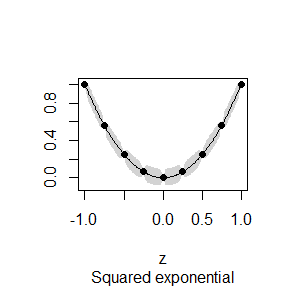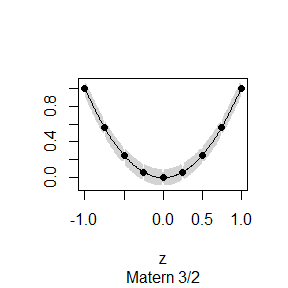## Constraints

Computational expense and numerical stability lead to some constraints on the type of data suitable for smooth supersaturated models. Due to the need for $$N>>n$$, larger dimensional datasets can be problematic in terms of memory and storage space for computation. Therefore fitting data with more than twenty variables may be time-consuming and inadvisable. For very low dimensional datasets, smooth supersaturated models are suitable for datasets with few points due to the numerical instability caused as the degree of terms in the polynomial basis get larger. It is difficult to set recommended values for $$N$$ as it is highly dependent on the number of variables and the design. As a rule of thumb, $$N=50$$ is an upper limit for one dimensional datasets, $$n=100$$ is generally suitable for two-dimensional datasets and $$n>200$$ should not be a problem for more variables. In general a value of $$N$$ should be sought that is as high as possible such that there is no instability visible in the main effects plot.

## Cross-validation

Cross-validation entails the use of the same model structure with many subsets of the full dataset. Rather than recompute the necessary objects each time (e.g. P, K, and so on) it is possible to pass a pre-existing SSM object to fit.ssm which will then use the same structure.

# ten point design in two factors
X <- matrix(runif(20, -1, 1), ncol = 2)
Y <- apply(X, 1, sum)
# fit SSM
s <- fit.ssm(X, Y);s
## Smooth supersaturated model in d=2 variables with N=50 terms over design of n=10 points.
## Smoothness measure Psi_2=2.09808e-29
# fit SSM with same structure to first nine design points only
s1 <- fit.ssm(X[1:9, ], Y[1:9], ssm = s);s1
## Smooth supersaturated model in d=2 variables with N=50 terms over design of n=9 points.
## Smoothness measure Psi_2=2.09634e-29